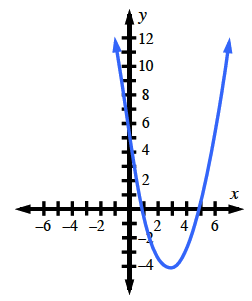### Home > CCA > Chapter 10 > Lesson 10.2.5 > Problem10-84

10-84.

Use your graphing shortcuts to graph $f(x)=x^2−6x+5$.

1. Where is the vertex?

Is the vertex the lowest or highest point on the graph?1. Describe the domain and range of this function.

Remember that the domain is the set of all inputs for which there is an output, and the range is the set of all possible outputs.
Look at the graph. Does the graph extend all the way left and right (domain)?
Does the graph extend all the way up and down (range)?

1. Does the vertex represent the maximum or minimum value of the function?

The vertex is the lowest/highest point on the graph.